Courses

# Test: SNAP Quant 2019

## 40 Questions MCQ Test Quantitative Aptitude (Quant) | Test: SNAP Quant 2019

Description
This mock test of Test: SNAP Quant 2019 for CAT helps you for every CAT entrance exam. This contains 40 Multiple Choice Questions for CAT Test: SNAP Quant 2019 (mcq) to study with solutions a complete question bank. The solved questions answers in this Test: SNAP Quant 2019 quiz give you a good mix of easy questions and tough questions. CAT students definitely take this Test: SNAP Quant 2019 exercise for a better result in the exam. You can find other Test: SNAP Quant 2019 extra questions, long questions & short questions for CAT on EduRev as well by searching above.
QUESTION: 1

### Solve this: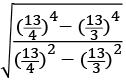Solution: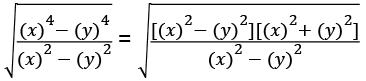Putting the values: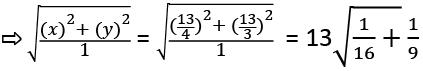13 x (5/12) = 65/12

=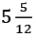QUESTION: 2

### What is the area of the shaded portion.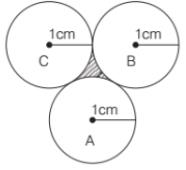Solution: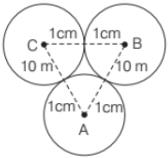∆ABC is an equilateral triangle of side 2 cm.

Area of an equilateral triangle = √3/4 x a2, where a is the side of that triangle.

Then, area of ∆ABC = √3/4x 2 x 2 = √3

Area of 3 sectors of angle 60° each and radius 1 cm each.

= 3 x π (radius)2 × (θ/360°)

= 3 x π (1)2 × (60°/360°) = π/2

Area of shaded portion = Area of ∆ABC – Area of 3 sectors

= √3 - (π/2)

QUESTION: 3

### Length of two trains is 150 m each. When they are moving in opposite directions. They cross each other in their 20s and when they are moving in the same direction. Then the faster train passed the slower train in the 40s. Then find the speed of the faster train.

Solution: Let speed of faster train = x m/s

Let speed of slower train = y m/s Distance = Speed × Time

Relative speed (in opposite direction) = (x + y) m/s

Relative speed (in same direction) = (x – y) m/s

In opposite direction

(x + y) = (150 + 150)/20 = 15 m/s

In same direction,

(x - y) = (150 + 150)/40 = 7.5 m/s

Using these two equations, we get x = 11.25 m/s

QUESTION: 4

Brigadier Rastogi travels from A to Bat 40 km/h on a bike. He travels from B to C at 10 km/h on cycle. The distance from A to Bisequal to B to C. Then he travels from C to A via B at 24 km/h by auto-rickshaw. Find his average speed.

Solution: Let distance from A to B LCM of 24, 10, 40 = 120 km

Time from A to B by bike = 120/40 = 3 h

Time from B to C by cycle = 120/10 = 12 h

All of us know that speed = Distance/time

Time from C to A by autorickshaw = 240/24 = 10 h

Average Speed = Total distance travelled/Total time taken

= (4 x 120)/(3 + 12 + 10) = 480/25 = 19.2 km/h

QUESTION: 5

Rohan and Rahul are 144 km apart on point A and point B respectively. Rohan travels constantly at 8 km/h. Rahul travels 4 km in the first hour, 5 km in the second hour, 6 km in the third hour and so on. Find the point where they well meet.

Solution: Speed of Rohan = 8 km/h

Speed of Rahul in start = 4 km/h and Rahul’s speed increases 1 km/h in every hour.

Relative speed in opposite direction AP series is formed = … 12, 13, 14 = Covered distance per hour

Let them meet after n hour.

According to the question, Distance between Rohan and Rahul = Covered distance in n hours Here, we will use the formula of sum of n terms of an A.P., which is:

S = n/2[2? + (? − 1)]

Where n = number of terms

a = First term of the A.P.

d = Common difference

144 = n/2[2 × 12 + (? − 1)1]

⇨ 288 = n(24 + n – 1)

Solving this equation,

n2 + 23n + 288 = 0

Splitting this quadratic equation, (n – 9) (n + 32) = 0

Here, we have two values of n, which are -32 and 9, but a negative value of n is not possible in this question.

So, after 9 h Rahul and Rohan meet, then distance covered by Rohan = 9 x 8 = 72 km.

It means, they meet exactly midway between A and B.

QUESTION: 6

Find the unit’s digit of  (1!)1! + (2!)2! + (3!)3! + …….. + (100!)100!.

Solution: 5! =  5 x 4 x 3 x 2 x 1 = 120

As we know, unit digit for (5!, 6!, 7!, 8! … ∞) is zero.

Also, (1!)1! + (2!)2! + (3!)3! + (4!)4! = 11 + 22 + 66 + 2424 .. . . . .. .(1)

Unit digit for (1): 1 + 4 + 6 + 6 = 17

∴ Required unit digit = 7.

QUESTION: 7

In how many ways can 10 books on mechanics and 8 books on quantum physics be placed in a row such that two books on quantum physics  may not be together?

Solution: Required ways = 11?8= (11 x 10 x 9)/(3 x 2 x 1) = 165

QUESTION: 8

In an institute, an MBA Exam is conducted and a sectional cut off has been introduced into it.  Any candidate appearing for the exam cannot qualify unless he clears the sectional cut off. If there are 4 sections in the paper, then what is the number of ways an applicant may fail in the exam.

Solution: There are two possibilities: pass (P) and Fail (F). Then, possibility of 4 sections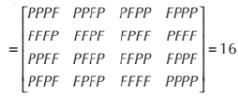Total possibility = 16

The number of ways on applicant may fail in the exam = (16 – 1) = 15

QUESTION: 9

In how many ways can letters of the word SOTICA be arranged such that vowels occupy odd positions?

Solution: SOTICA has three vowels. Then, the required ways = 3! x 3! = 6 x 6 = 36

QUESTION: 10

Square, Circle, Hexagon and Octagon have equal perimeter. Which has the maximum area?

Solution: If the perimeter of figures is the same then, the area of the figure which has the maximum number of sides is maximum. Then, the circle has a maximum area.

QUESTION: 11

In how many ways can one wrap 3 Kitkat, 2 Fivestar and 3 Bar one. If at least one Kitkat has to be there in the gift pack and the gift pack has three chocolates.

Solution: Number of ways of the gift pack = 8?3 = 56

Number of ways of the gift pack without Kitkat = 8?3 = 10

Then, number of ways of the gift pack with Kitkat = 56 – 10 = 46

QUESTION: 12

The difference between compound and simple interest for a loan is 114 when invested for 2 yr. The rate of interest is 6% per annum. Find the loan amount.

Solution: Difference between compound and simple interest for 2 years of a principal P with interest rate r% per annum = P(r/100)2

Using this formula:

114 = P(6/100)2

⇨ P = (114 x 100 x 100)/(6 x 6) = 31666.66

QUESTION: 13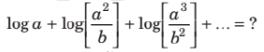Solution: Using the properties of Log, and simplifying this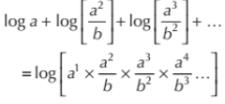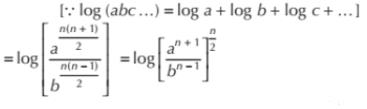QUESTION: 14

What is the coefficient of z3 in -7x y2 z3a2b2.

Solution: The given polynomial is -7x y2 z3a2b2

If we take z3 out of this, it becomes: z3 (-7x y2 z3a2b2

Hence, (-7x y2 z3a2b2) is the coefficient of z3 in the given polynomial.

QUESTION: 15

In a regular hexagon field, ropes are tied to connect all vertices (diagonal and side). What is the number of intersection points of ropes?

Solution: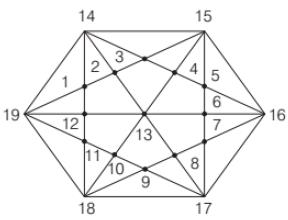We can see in the above figure that there are 19 intersection points.

QUESTION: 16

In the given diagram, ∠ CAB = 60° and BC = a, AC = b, and AB = c.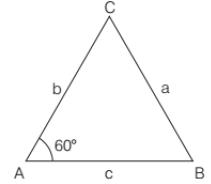Then which of the following is correct?

Ans. b

Solution: Here, we are using “Cosine rule”, which is as follows

Cos 60° = (b2 + c2– a2)/2bc

Putting the value of Cos 60°, which is 1/2 and doing the cross multiplication.

a2 = b2+ c2 – bc

QUESTION: 17

In a closed wooden box, length = 20 cm breadth = 14 cm and height = 10 cm and thickness = 5 mm. If the weight of an empty box is 3.462 kg, then what is the weight of 1 cm 3 of wood (in gms)?

Solution: Volume of cuboid = Length × Breadth × Height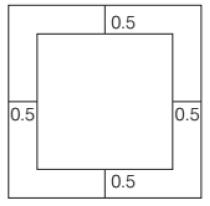Volume of wood = External volume of box with wood – Internal volume of box without wood

= (LBH)external – (LBH)internal

= 20 x 14 x 10 = 19 x 13 x 9

= 577 cm3

Total weight of wood = 3.462 kg

Weight of 577 cm3 of wood = 3462 gm

Then, weight of 1 cm3 of wood cm3 = 3462/577 = 6 gms

QUESTION: 18

Find the number of zeros at the end of (5!)5! + (10!)10! + (50!)50! + (100!)100!

Solution: (5!)5!  is added to the remaining numbers then only we have to find the number of zero in (5!)5!

5! = 5 x 4 x 3 x 2 x 1 = 120

Now, 120120 will have 120 zeroes.

QUESTION: 19

Gitesh is twice as good as Jitesh. Gitesh takes 30 days less than Jitesh to finish a task. How long will Gitesh and Jitesh take to complete the task together.

Solution: Let efficiency of Gitesh = 2x, and efficiency of Jitesh = x

Jitesh is half efficient as Gitesh, so he will take double time to finish the task.

And, the difference between their time is 30 days.

So, Jitesh’s time = 2 x 30 = 60 days

And, Gitesh’s time = x = 30 days

Let us assume the total work to be 60 units.

Jitesh will do 1 unit per day.

Gitesh will do 2 units per day.

Together they will do 3 units per day.

Time taken by both, when working together = 60/3 = 20 days.

QUESTION: 20

Mohan has a 200 L container. K L of milk is kept in the container. Mohan removes 6L milk and adds 6L water. He again replaces 6L of solution with water. Now milk and water are in the ratio 9: 16. What is the value of K?

Solution: Water which was added =

Initial quantity of substance x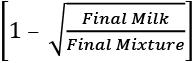Putting the values: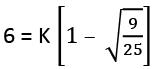⇨ 6 = 2K/5

⇨ K = 15 litres

QUESTION: 21

Indian express travels at 60 km/h and halts. For fixed time every hour. Due to halts, the average speed becomes 50 km/h. Find the time of halt.

Solution: Halt time = [(Faster train’s speed – Slower train’s speed)/Faster train’s speed] x 60

[(60 – 50)/60] x 60 = 10 mins.

QUESTION: 22

Directions: Read the given information carefully and answer the given question. In 2015, 100 aspirants appeared for an exam.

The number of students who qualified in Maths = 55.

The number of students who qualified in LR= 38.

The number of students who qualified in (Maths + English) = 30.

The number of students who qualified in (LR + English) = 15.

The number of students who qualified in (Maths + LR) = 20.

The number of students who qualified in (Maths + LR + English) = 5.

The number of students who qualified in DI = 22.

The number of students who qualified in (DI + LR) = 5.

The number of students who qualified in (DI + Maths) = 5.

The number of students who qualified in (DI + Maths + LR) = 5.

The number of students who qualified in English = 50.

Those who qualified in English, could not qualify in the DI section.

Q. How many qualified at least two sections?

Solution: Using the Venn Diagram concepts, we can draw a diagram like this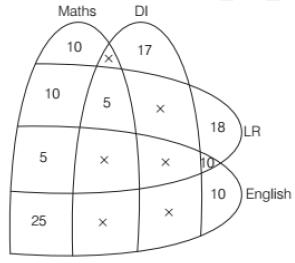Qualified at least two section = 10 + 5 + 5 + 25 + 10 = 55 aspirants.

QUESTION: 23

Directions: Read the given information carefully and answer the given question. In 2015, 100 aspirants appeared for an exam.

The number of students who qualified in Maths = 55.

The number of students who qualified in LR= 38.

The number of students who qualified in (Maths + English) = 30.

The number of students who qualified in (LR + English) = 15.

The number of students who qualified in (Maths + LR) = 20.

The number of students who qualified in (Maths + LR + English) = 5.

The number of students who qualified in DI = 22.

The number of students who qualified in (DI + LR) = 5.

The number of students who qualified in (DI + Maths) = 5.

The number of students who qualified in (DI + Maths + LR) = 5.

The number of students who qualified in English = 50.

Those who qualified in English, could not qualify in the DI section.

Q. How many qualified in both Maths and LR, but not in any other section?

Solution: Using the Venn Diagram concepts, we can draw a diagram like thisQualified in both Maths and LR = 10 aspirants.

QUESTION: 24

Directions: Read the given information carefully and answer the given question. In 2015, 100 aspirants appeared for an exam.

The number of students who qualified in Maths = 55.

The number of students who qualified in LR= 38.

The number of students who qualified in (Maths + English) = 30.

The number of students who qualified in (LR + English) = 15.

The number of students who qualified in (Maths + LR) = 20.

The number of students who qualified in (Maths + LR + English) = 5.

The number of students who qualified in DI = 22.

The number of students who qualified in (DI + LR) = 5.

The number of students who qualified in (DI + Maths) = 5.

The number of students who qualified in (DI + Maths + LR) = 5.

The number of students who qualified in English = 50.

Those who qualified in English, could not qualify in the DI section.

Q. How many did not qualify any section?

Solution: Using the Venn Diagram concepts, we can draw a diagram like thisNone of the students failed i.e., 0.

QUESTION: 25

Directions: Read the given information carefully and answer the given question. In 2015, 100 aspirants appeared for an exam.

The number of students who qualified in Maths = 55.

The number of students who qualified in LR= 38.

The number of students who qualified in (Maths + English) = 30.

The number of students who qualified in (LR + English) = 15.

The number of students who qualified in (Maths + LR) = 20.

The number of students who qualified in (Maths + LR + English) = 5.

The number of students who qualified in DI = 22.

The number of students who qualified in (DI + LR) = 5.

The number of students who qualified in (DI + Maths) = 5.

The number of students who qualified in (DI + Maths + LR) = 5.

The number of students who qualified in English = 50.

Those who qualified in English, could not qualify in the DI section.

Q. How many qualified only DI sections?

Solution: Using the Venn Diagram concepts, we can draw a diagram like thisQualified only in DI = 17.

QUESTION: 26

Directions: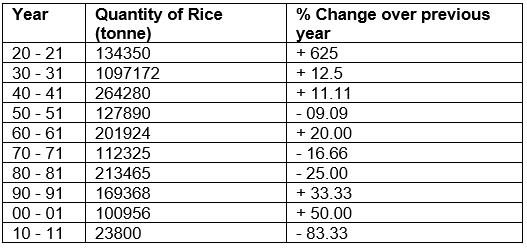Q. What is the approximate production of rice in the year 1949-50?

Solution: Production of rice in year 1950 – 51= 127890 tonne

Production of rice in 1950-51 is 09.09% less than that of in the year 1949-50.

As we know that 09.09% is 1/11. Using it below:

Then, production of rice in 1949-50

= (11/10) x Production of rice in year 1950-51

= (11/10) x 127890 = 140679 tonne

QUESTION: 27

Directions:Q. What is the difference in the production of rice in 1969-70 and 1979-80?

Solution: Required difference = (Production of rice in 1979-80) − (Production of rice in 1969-70) Using the fraction values of %: = 213465 x (4/3) – 112325 x (6/5) = 284620 – 134790 = 149830 tonne.

QUESTION: 28

Directions:Q. What is the approximate production in 1959-60?

Solution: Production of rice in 1960-61= 201924 tonne

The production in 1959-60:

5/6 of 201924 = 168270 tonne

QUESTION: 29

What is the sum of integers from 113 to 113113, which are divisible by 7?

Solution: 119 + 126 + …….. + 113113

This is the series of AP.

Let the total number of terms be n.

Last number of an AP = a + (n – 1)d

Where a = first term

n = number of terms

d = common difference

Putting the values in the formula:

113113 = 119 + (n – 1) x 7

n = 16143

There is another formula, and it is for the sum of n terms of an AP when first and last terms are given.

Sum = n/2(a + l)

= 16143/2 x (119 + 113113)

= 913952088

QUESTION: 30

Robot is 4 m in length and placed at a corner of a 16 m × 30 m field. The robot is facing a diagonally opposite corner and reaches the diagonally opposite corner in 15 s. What is the speed (in m/s) of a robot?

Solution: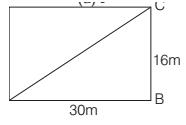ABCD is rectangle. Then, ∠B = 90°

Using the Pythagorean Theorem:

AC = √256 + √900 = √1156 = 34

Length of the Robot = 4 m.

Travelled distance by the Robot = 34 – 4 = 30 m

Speed of the Robot = 30/15 = 2 m/s

QUESTION: 31

In a smart phone factory, Robot A, B, and C manufacture 25%, 35% and 40% circuit boards respectively. for each of the robot faulty circuit boards = 5%, 4% and 2% respectively. What is the probability that the printed circuit board is defective if 1 circuit board is picked at random?

Solution: Let total circuit board manufacture in factory = 100k

Then, the circuit board manufactured by the Robots:

A = 100k (25/100) = 25k

B = 100k (35/100) = 35k

C = 100k (40/100) = 40k

Number of faulty circuit boards by Robots:

A = 25k (5/100) = 1.25k

B = 35k (4/100) = 1.4k

C = 40k (2/100) = 0.8k

Total faulty boards = 3.45k

Probability = 3.45k/100k = 0.034

QUESTION: 32

Average stipend of a group of students is 50 per day. The difference between maximum and minimum stipend is 45. If these 2 students are excluded, the average decreases by 1. Minimum earnings lie between 42 to 47 and the number of students is a prime number, where both the digits are also prime. What is the number of students initially?

Solution: Let the minimum stipend = x

Then, the maximum stipend = x + 45

Let number of students = n

Then, total stipend of a group of students = 50n …(i)

New average = 49

Then, total stipend of a group of students = 49 (n – 2) ….. (ii)

By Equations. (i) and (ii), we get the stipend of two students

50n - 49 (n – 2) = n + 98

As per the question, n + 98 = x + x + 45

Minimum earnings lie between 42 to 47. Putting x = 45.

n + 98 = 2(45) + 45

n = 37

For x = 45, n is a prime number. Number of students = 37.

QUESTION: 33

Valve A fills a bathtub in 10 h and Valve B fills the bathtub in 15 h. When A and B are opened together, later B closes after some time. Total time taken to fill the bathtub is 5h. After how much time was B closed?

Solution: Valve A fills a bathtub = 10 h

Valve B fills the bathtub = 15 h

Let us assume the capacity of the bathtub = 150 litres

A’s 1hour filling work = 15 litres

B’s 1hour filling work = 10 litres

A was on for 5 hours, it would have filled 15 x 5 = 75 litres.

Remaining 75 litres were filled by A + B, time taken by them to fill this: 75/(15 + 10) = 3 h

B was on for 3 hours.

QUESTION: 34

Directions: Refer to the pie-chart and answer the given questions.

Percentage of employees in different departments of branch ‘XYZ’ in the year 2014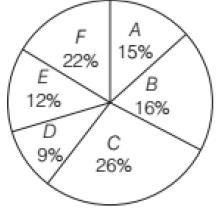Total number of employees = 450

Q. In 2014, the number of female employees in department C was 5/13 of the total number of employees in the same department. If the number of female employees in department F was 4 less than that in department C, what is the number of male employee’s department F?

Solution: Total number of employees in department C

450 x 0.26 = 117

Female employees in department C = 117 x (5/13) = 45

Now, total number of employees in department F = 450 x (22/100) = 99

Female employees in department F = 45 – 1 = 41

Male employees in F department = 99 – 41 = 58

QUESTION: 35

Directions: Refer to the pie-chart and answer the given questions.

Percentage of employees in different departments of branch ‘XYZ’ in the year 2014Total number of employees = 450

Q. The number of employees in department E is what percent less than the number of employees in departments A and C together?

Solution: As per the pie chart, E has 12%.

A and C together have 26 + 15 = 41%

Required Percentage = [(41 – 12)/41] x 100 = 70% (Approximately)

QUESTION: 36

Directions: The following pie chart shows the analysis of the result of an examination in which 5 candidates have failed. Study the chart and answer the questions given below.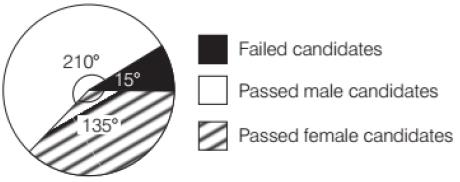Q. The total number of examinees was

Solution: Full circle pie chart makes the angle of 360.

15 degrees is for failed students, and as per the question, there are 5 failed students.

15 degrees means 5 students.

360 degree means (5/15) x 360 = 120.

QUESTION: 37

Directions: The following pie chart shows the analysis of the result of an examination in which 5 candidates have failed. Study the chart and answer the questions given below.Q. Percentage of passed female candidates with respect to total examinees is

Solution: 135 degree is for passed female candidates.

Calculating the percentage = (135/360) x 100 = 37.5%

QUESTION: 38

Directions: The following pie chart shows the analysis of the result of an examination in which 5 candidates have failed. Study the chart and answer the questions given below.Q. Percentage of passed male candidates with respect to total passed candidates is

Solution: 210 degree is for passed male candidates.

Percentage of passed male candidates with respect to total passed candidates is: (210/345) x 100 = 60.8 %

QUESTION: 39

Directions: The following pie chart shows the analysis of the result of an examination in which 5 candidates have failed. Study the chart and answer the questions given below.Q. Ratio of passed male candidates to the successful female candidates is

Solution: Ratio of passed male candidates to the successful female candidates is: 210/135 = 14 : 9

QUESTION: 40

Find the value of m – n, if

9n x 32 x (3-n / 2)-2 – (27)n / 33m x 23 = 1 / 27

Solution:

Simplifying for n, and using the properties of powers

9n x 32 x (3-n / 2)-2 – (27)n / 33m x 23 = 1 / 27

32n x 32 – 3n- 33n / 33m x 23 = 1 / 27

32n+2 - 33n / 33m x 8 = 1 / 27

33n(32 – 1) / 33m x 8 = 1 / 27

(33)n – m = 3-3

n – m = -1 or m – n = 1